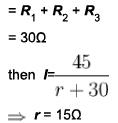Courses

# Semiconductor Diode NAT Level - 2

## 10 Questions MCQ Test Topic wise Tests for IIT JAM Physics | Semiconductor Diode NAT Level - 2

Description
This mock test of Semiconductor Diode NAT Level - 2 for Physics helps you for every Physics entrance exam. This contains 10 Multiple Choice Questions for Physics Semiconductor Diode NAT Level - 2 (mcq) to study with solutions a complete question bank. The solved questions answers in this Semiconductor Diode NAT Level - 2 quiz give you a good mix of easy questions and tough questions. Physics students definitely take this Semiconductor Diode NAT Level - 2 exercise for a better result in the exam. You can find other Semiconductor Diode NAT Level - 2 extra questions, long questions & short questions for Physics on EduRev as well by searching above.
*Answer can only contain numeric values
QUESTION: 1

### Calculate form factor of halfwave rectifier as a multiple of π ?

Solution:

The ratio of rms value to the average value is called form factor. Thus,
form factor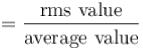for half wave rectifier, lrms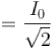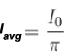form factor =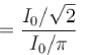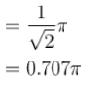*Answer can only contain numeric values
QUESTION: 2

### Calculate the zener current in the given circuit in mA?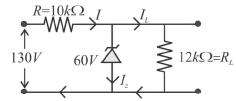Solution: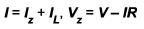and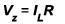Now 130-60 = IR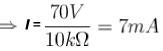Now, Vz = lLRL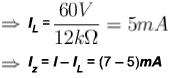Iz = 2mA

*Answer can only contain numeric values
QUESTION: 3

### A 24V, 600mW, zener diode is to be used in for providing a 24 Vstabilized supply to variable load.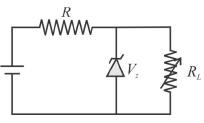Assuming for a proper zener action, a minimum of 10mA must flow through the zener. If the input voltage is 32 V. What would be the value of RL (in ohm).

Solution: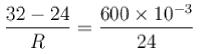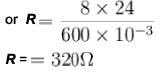*Answer can only contain numeric values
QUESTION: 4

What is the potential difference across R1 (in volts) ?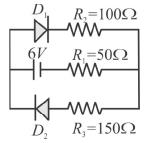Solution:

D2 is reverse biased, hence, no current flow through it.
6 - iR2 - iR1=0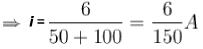Now potential difference across R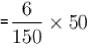= 2V

*Answer can only contain numeric values
QUESTION: 5

The 6 Volts zener diode shown in figure has zero zener resistance and knee current of 5mA. The minimum value of R (in Ω), so that the voltage across it does not fall below 6 Volts is ?

Solution: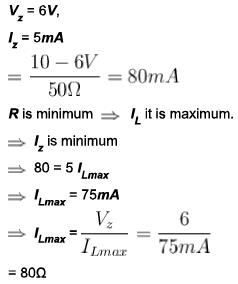*Answer can only contain numeric values
QUESTION: 6

Current 2A and 1A flow when large forward voltage V1 and V2 are applied to a semiconductor diode. If V1, = V2, then the value of the reverse saturation current is. (in A)

Solution: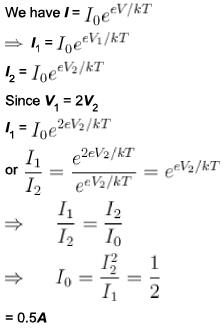*Answer can only contain numeric values
QUESTION: 7

The centre tap full-wave single phase rectifier circuit uses 2 diodes as shown in the given figure. The rms voltage across each diode is (in Volts)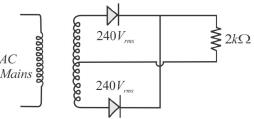Solution:

Peak inverse voltage (PIV) across each diode =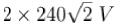when diode is conducting (during half cycle) the voltage across the diode is zero.
Hence, rms value of voltage across the diode =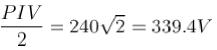*Answer can only contain numeric values
QUESTION: 8

A half-wave rectifier employs a transformer with turn ratio n1 : n= 12 : 1. If primary coil is connected to the power mains 220V, 50Hz. If the diode resistance in forward bias is negligible, what is the peak inverse voltage (in Volts) of the diode?

Solution:

The turn ratio of transform ation is 12:1. So if primary coil is connected to power mains o f 220V , then potential of secondary coil is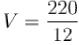Volts. In a half-wave rectifier,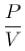is the maximum voltage appearing across the transformer secondary coil. Now. peak inverse voltage,for half wave rectifier in given as V0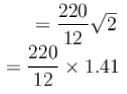= 25.9V
The correct answer is: 25 .9

*Answer can only contain numeric values
QUESTION: 9

A 10V zener diode working as a voltage regulator operates from a source that varies from 5 V to 15V, the series resistance is 0.1kΩ and the load draws a current that varies from 0 to 20mA. The power dissipation in the zener diode under worst case (in Watts) is?

Solution: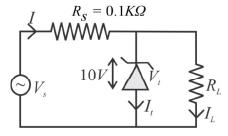For maximum power dissipation, current through zener diode should be maximum which is maximum when total current in the circuit is maximum i.e. Vs = 15V.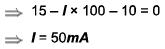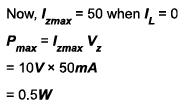*Answer can only contain numeric values
QUESTION: 10

When three zener diodes of identical specification having 10W, 10 V, l000 mA are connected in series to a 45V~dc power supply. Calculate the series resistance required to obtain 30 V regulated output (in Ω)?

Solution:

Power of each diode = 10W
Potential = 10
⇒ current = 1A
⇒ Resistance of each zener diode R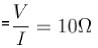⇒ Equivalent resistance of three diodes connected in series